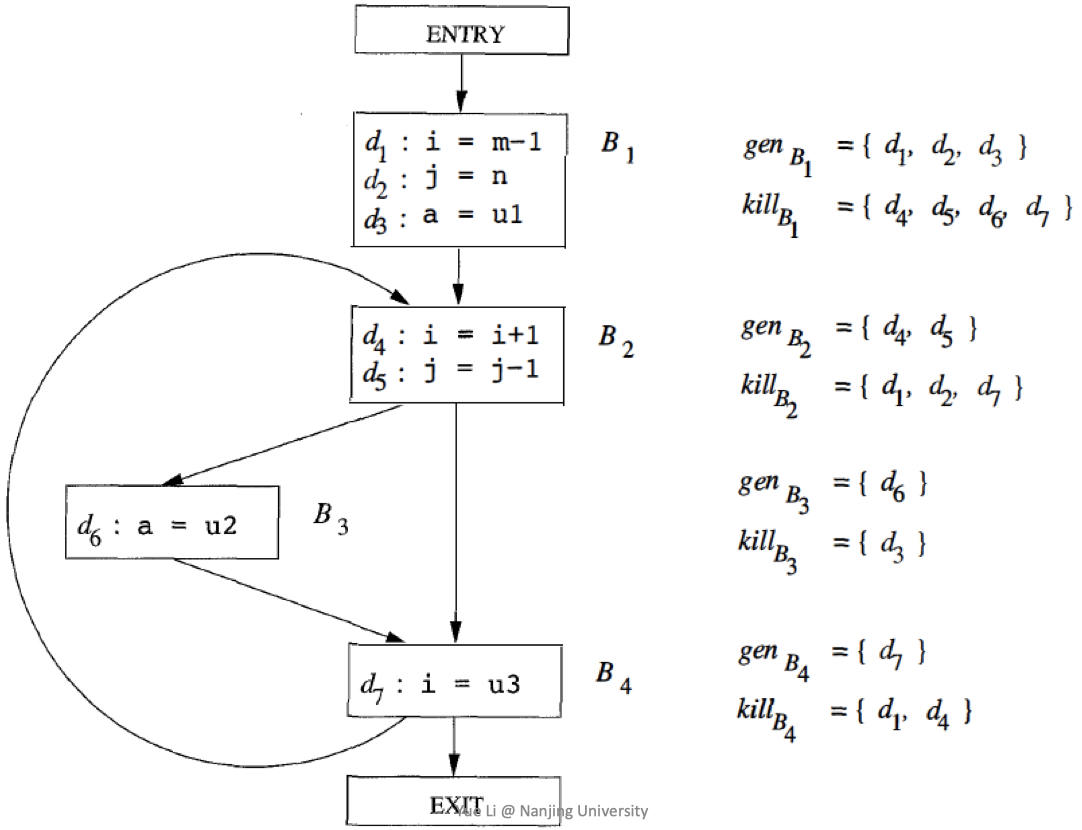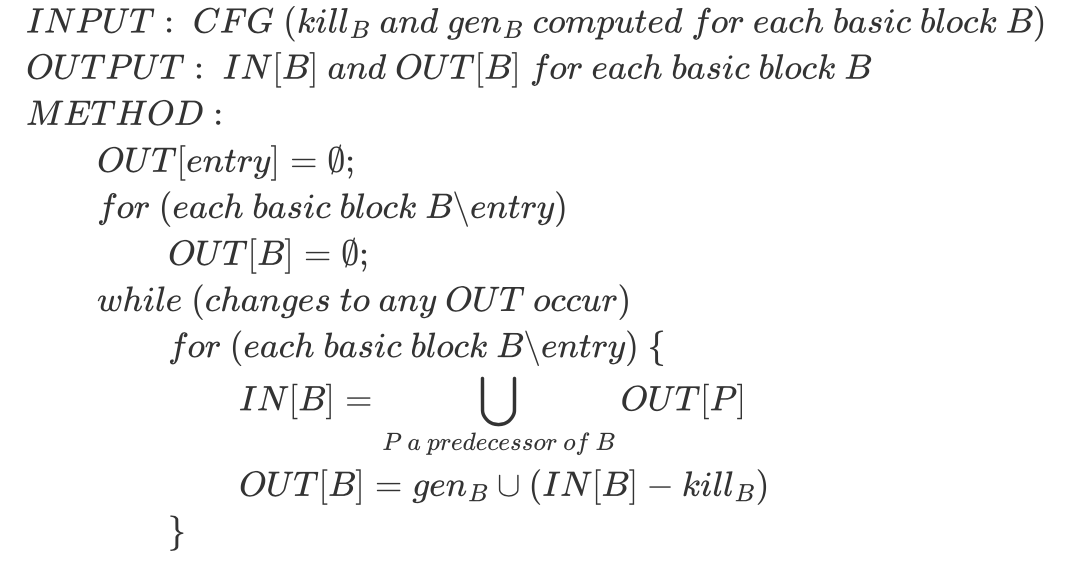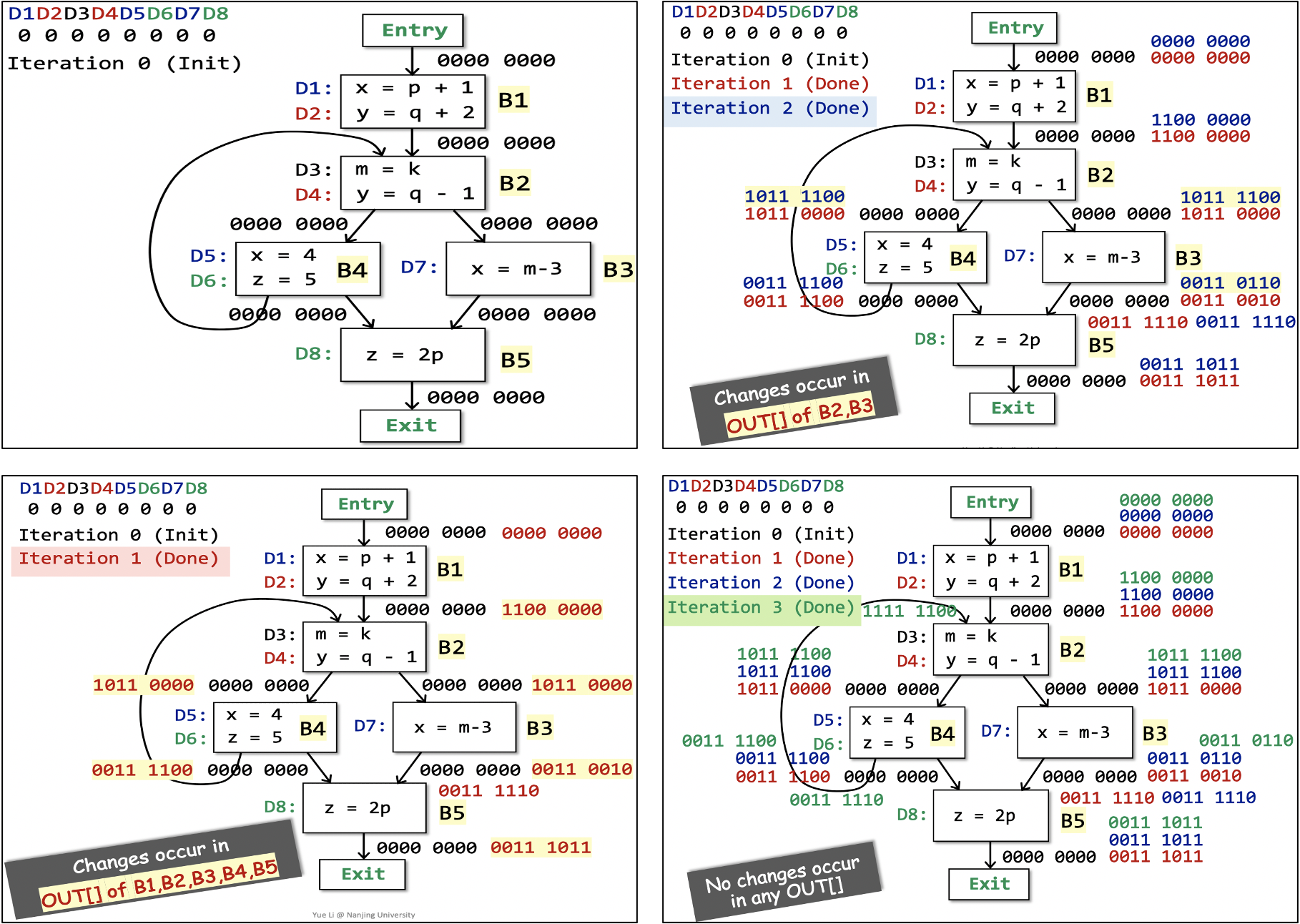## 数据流分析基础

• 每个IR语句的执行将一个输入状态（input state）转换成了一个新的输出状态（output state）。
• 某个IR语句的输入（输出）状态与该语句之前（之后）的程序点（program point）关联。

IN[s1]   │              ┌──────────┐       ┌──────────┐      ┌──────────┐
│              │          │       │          │      │          │
┌────┴─────┐        │    s1    │       │    s1    │      │    s3    │
│          │        │          │       │          │      │          │
│    s1    │        └─────┬────┘       └────┬─────┘      └─────┬────┘
│          │              │                 │                  │
└────┬─────┘          ┌───┴──┐              └────────┬─────────┘
OUT[s1]  │                │      │                       │
▼                ▼      ▼                       ▼
┌──────────┐ ┌──────────┐   ┌──────────┐        ┌──────────┐
│          │ │          │   │          │        │          │
│    s2    │ │    s2    │   │    s3    │        │    s2    │
│          │ │          │   │          │        │          │
└──────────┘ └──────────┘   └──────────┘        └──────────┘


• 左侧控制流，IN[s2] = OUT[s1]
• 中间控制流，IN[s2] = IN[s3] = OUT[s1]
• 右侧控制流，IN[s2] = OUT[s1] ^ OUT[s3]

^是meet operator，根据实际控制流走向取值。

1. 基于语句语义的约束（表示为transfer functions）。
2. 基于控制流的约束。

1. 前向分析（forward analysis），根据输入求输出：$OUT[s] = f_{s}(IN[s])$
2. 后向分析（backward analysis），根据输出求输入：$IN[s] = f_{s}(OUT[s])$

1. BB内控制流：$IN[s_{i+1}] = OUT[s_{i}],\ for\ all\ i = 1, 2, …, n-1$
2. BB间控制流：$IN[B] = IN[s_{1}],\ OUT[B] = OUT[s_{n}]$

• $OUT[B] = f_{B}(IN[B]), f_{B} = f_{s_{n}} \circ\ …\ \circ f_{s_{2}} \circ f_{s_{1}}$
• $IN[B] = \bigwedge_{P\ a\ predecessor\ of\ B} OUT[P]$

$\bigwedge\$符号用于表示B的所有前驱对B的贡献的综合结果。

• $IN[B] = f_{B}(OUT[B]), f_{B} = f_{s_{1}} \circ\ …\ \circ f_{s_{n-1}} \circ f_{s_{n}}$
• $OUT[B] = \bigwedge_{S\ a\ successor\ of\ B} IN[S]$

## Reaching Definitions Analysis

• 函数调用，涉及到跨过程的CFG。
• 别名（Aliases），目前我们处理的都是没有别名的变量。

OK，首先看一下“定义可达性”这个概念：

A definition d at program point p reaches a point q if there is a path from p to q such that d is not “killed” along that path.1. 为什么 $OUT[entry] = \empty$ 被单独拎出来了，没有和下面的for循环合并？
2. 为什么我们能确定while循环一定会终止？

OK，接下来看一个例子：